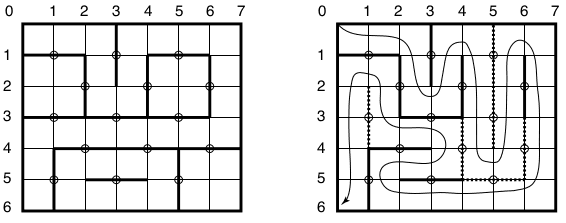### F - Fix the Pond

##### Languages: C, C++, Java, Pascal, ... (details)

A shrimp farm uses a rectangular pond built as a grid with $2N$ rows and $2N + 1$ columns of square cells, for a given integer $N$. Each cell side is one meter long. The pond has exactly $(2N - 1) \times N$ barriers of length two meters, used to temporarily isolate smaller sections inside the pond for breeding different kinds of shrimp. The barriers have their middle points fixed precisely at the integer coordinates $(a, b)$, for all $0 \lt a \lt 2N$ and $0 \lt b \lt 2N + 1$, where both a and b are odd, or both are even. Each barrier can be rotated around its middle point to change the pond configuration; however, by being rotated, a barrier switches between only two possible positions, always parallel to the pond sides, vertical or horizontal. The left part of the figure below shows a pond configuration, with $N = 3$.At the end of every season the pond is closed for maintenance and cleaning. It must then be reconfigured so that a special machine can sweep the pond floor. The machine starts its work at the top left cell, and needs to pass through every cell exactly once, finishing in the bottom left cell. The right part of the figure shows one such reconfiguration, where six barriers were switched. For this example, though, four barrier switches would have been enough.

You must write a program that given a pond configuration, determines the minimum number of barrier switches needed to reconfigure the pond as specified above. There is always at least one possible way to reconfigure the pond as specified.

#### Input

Each test case is described using several lines. The first line contains an integer $N$ indicating that the pond has $2N$ rows and $2N + 1$ columns $(1 \leq N \leq 300)$. Each of the next $2N - 1$ lines contains a string of $N$ characters describing the orientation of the barriers. In the $i$-th line, the $j$-th character indicates the orientation of the barrier whose middle point is at coordinates $(i, 2j - 1)$ if i is odd, or $(i, 2j)$ if i is even, for $i = 1, 2, ..., 2N - 1$ and $j = 1, 2, ..., N$. The character is the uppercase letter $\texttt{V}$ if the orientation is vertical, or the uppercase letter $\texttt{H}$ if it is horizontal.

#### Output

For each test case output a line with an integer representing the minimum number of barrier switches needed to reconfigure the pond as specified.

#### Sample test(s)

Input
3 HVH VVV HHH HHH VHV 1 H 1 V
Output
4 0 1